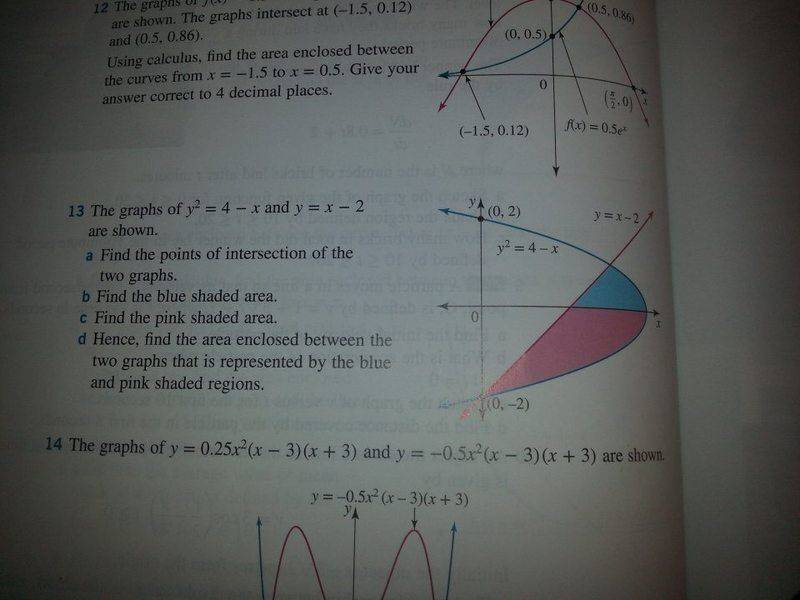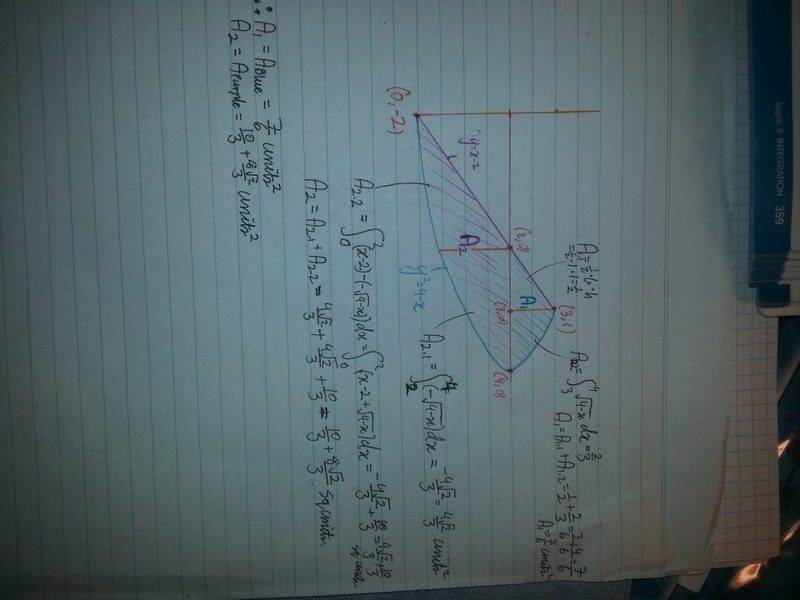# Area enclosed between y^2=4-x and y=x-2

## Homework Equations

[/B]
Definite integrals and area between two curves

## The Attempt at a Solution

[/B]
Also find attached.

The answer in the back of the book says that part c should be 10/3, but that would mean that (8sqrt (2))/3 would need to cancel out in both area 2.1 and area 2.2, whichdoesn't work because areas are always positive.fresh_42
Mentor
2021 Award
Why didn't you calculate the area under the parabola and only subtract the triangle? It seems you used more areas than necessary. But I'm not good at reading on the side.

Why didn't you calculate the area under the parabola and only subtract the triangle? It seems you used more areas than necessary. But I'm not good at reading on the side.
Ah yes that is a much better idea thank you. But still, my method should still be getting the same answer but it's not. Could you please explain to me where I'm going wrong?

SteamKing
Staff Emeritus
Homework Helper

## Homework Equations

[/B]
Definite integrals and area between two curves

## The Attempt at a Solution

[/B]
Also find attached.

The answer in the back of the book says that part c should be 10/3, but that would mean that (8sqrt (2))/3 would need to cancel out in both area 2.1 and area 2.2, whichdoesn't work because areas are always positive.
View attachment 101868 View attachment 101869
Areas may always be positive, but definite integrals are not required to be positive. To calculate an area using a definite integral sometimes requires some interpretation of what the definite integral means in that case.

http://tutorial.math.lamar.edu/Classes/CalcI/AreaBetweenCurves.aspx

Going off fresh_42's comment, you can also evaluate the definite integral to calculate the area under a curve using dy instead of dx as the variable of integration.

fresh_42
Mentor
2021 Award
Ah yes that is a much better idea thank you. But still, my method should still be getting the same answer but it's not. Could you please explain to me where I'm going wrong?
To be honest: I didn't like the root expressions. First thing I did was to exchange x and y.

Mark44
Mentor
Going off fresh_42's comment, you can also evaluate the definite integral to calculate the area under a curve using dy instead of dx as the variable of integration.
In other words, using horizontal strips instead of vertical strips.

Thank you all for your help, but I feel like some of this stuff is a little beyond my current course. We've only just started looking at definite integrals, and have spent even less time with areas between two curves. I highly doubt we're expected to be using horizontal strips instead of vertical ones when we haven't even learned about conics or anything really.

But again thank you all for your help still

SteamKing
Staff Emeritus
Homework Helper
Thank you all for your help, but I feel like some of this stuff is a little beyond my current course. We've only just started looking at definite integrals, and have spent even less time with areas between two curves. I highly doubt we're expected to be using horizontal strips instead of vertical ones when we haven't even learned about conics or anything really.

But again thank you all for your help still
You don't need to know anything about conics to switch the variable of integration. Often times, switching is done as a matter of convenience to simplify the integral.

I think my main problem is that I still don't know how to calculate the area between two curves properly.

Say I was just given y=x-2 and y=-sqrt(4-x) and had to find the area enclosed between them between x=0 and x=2
Isn't the correct way of doing this to just set up an integral over the given domain and subtract the function on the bottom from the function in top? So integral(2, 0, (x-2-(-sqrt(4-x)))dx

fresh_42
Mentor
2021 Award
You don't need to know anything about conics to switch the variable of integration. Often times, switching is done as a matter of convenience to simplify the integral.
.... or simply exchange x and y and integrate as you are used to, which is the same.

.... or simply exchange x and y and integrate as you are used to, which is the same.
Okay thank you I'll just do it that way